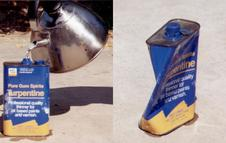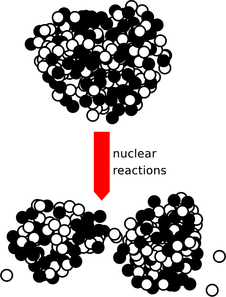$$\require{cancel}$$

# 2.4 Atomic Phenomena

•• Contributed by Benjamin Crowell
• Professor (Physics) at Fullerton College

Variety is the spice of life, not of science. So far this chapter has focused on heat energy, kinetic energy, and gravitational energy, but it might seem that in addition to these there is a bewildering array of other forms of energy. Gasoline, chocolate bars, batteries, melting water --- in each case there seems to be a whole new type of energy. The physicist's psyche rebels against the prospect of a long laundry list of types of energy, each of which would require its own equations, concepts, notation, and terminology. The point at which we've arrived in the study of energy is analogous to the period in the 1960's when a half a dozen new subatomic particles were being discovered every year in particle accelerators. It was an embarrassment. Physicists began to speak of the “particle zoo,” and it seemed that the subatomic world was distressingly complex. The particle zoo was simplified by the realization that most of the new particles being whipped up were simply clusters of a previously unsuspected set of fundamental particles (which were whimsically dubbed quarks, a made-up word from a line of poetry by James Joyce, “Three quarks for Master Mark.”) The energy zoo can also be simplified, and it's the purpose of this section to demonstrate the hidden similarities between forms of energy as seemingly different as heat and motion.

# 2.4.1 Heat is kinetic energy.

What is heat really? Is it an invisible fluid that your bare feet soak up from a hot sidewalk? Can one ever remove all the heat from an object? Is there a maximum to the temperature scale?

The theory of heat as a fluid seemed to explain why colder objects absorbed heat from hotter ones, but once it became clear that heat was a form of energy, it began to seem unlikely that a material substance could transform itself into and out of all those other forms of energy like motion or light. For instance, a compost pile gets hot, and we describe this as a case where, through the action of bacteria, chemical energy stored in the plant cuttings is transformed into heat energy. The heating occurs even if there is no nearby warmer object that could have been leaking “heat fluid” into the pile.

An alternative interpretation of heat was suggested by the theory that matter is made of atoms. Since gases are thousands of times less dense than solids or liquids, the atoms (or clusters of atoms called molecules) in a gas must be far apart. In that case, what is keeping all the air molecules from settling into a thin film on the floor of the room in which you are reading this book? The simplest explanation is that they are moving very rapidly, continually ricocheting off of the floor, walls, and ceiling. Though bizarre, the cloud-of-bullets image of a gas did give a natural explanation for the surprising ability of something as tenuous as a gas to exert huge forces.a / A vivid demonstration that heat is a form of motion. A small amount of boiling water is poured into the empty can, which rapidly fills up with hot steam. The can is then sealed tightly, and soon crumples.

The experiment shown in figure a, for instance, can be explained as follows. The high temperature of the steam is interpreted as a high average speed of random motions of its molecules. Before the lid was put on the can, the rapidly moving steam molecules pushed their way out of the can, forcing the slower air molecules out of the way. As the steam inside the can thinned out, a stable situation was soon achieved, in which the force from the less dense steam molecules moving at high speed balanced against the force from the more dense but slower air molecules outside. The cap was put on, and after a while the steam inside the can began to cool off. The force from the cooler, thin steam no longer matched the force from the cool, dense air outside, and the imbalance of forces crushed the can.b / Random motion of atoms in a gas, a liquid, and a solid.

This type of observation leads naturally to the conclusion that hotter matter differs from colder in that its atoms' random motion is more rapid. In a liquid, the motion could be visualized as people in a milling crowd shoving past each other more quickly. In a solid, where the atoms are packed together, the motion is a random vibration of each atom as it knocks against its neighbors.

We thus achieve a great simplification in the theory of heat. Heat is simply a form of kinetic energy, the total kinetic energy of random motion of all the atoms in an object. With this new understanding, it becomes possible to answer at one stroke the questions posed at the beginning of the section. Yes, it is at least theoretically possible to remove all the heat from an object. The coldest possible temperature, known as absolute zero, is that at which all the atoms have zero velocity, so that their kinetic energies, $$K=(1/2)mv^2$$, are all zero. No, there is no maximum amount of heat that a certain quantity of matter can have, and no maximum to the temperature scale, since arbitrarily large values of $$v$$ can create arbitrarily large amounts of kinetic energy per atom.

The kinetic theory of heat also provides a simple explanation of the true nature of temperature. Temperature is a measure of the amount of energy per molecule, whereas heat is the total amount of energy possessed by all the molecules in an object.

There is an entire branch of physics, called thermodynamics, that deals with heat and temperature and forms the basis for technologies such as refrigeration. Thermodynamics is discussed in more detail in chapter 5, and I've provided here only a brief overview of the thermodynamic concepts that relate directly to energy.

# 2.4.2 All energy comes from particles moving or interacting.

If I stretch the spring in figure c and then release it, it snaps taut again. The creation of some kinetic energy shows that there must have been some other form of energy that was destroyed. What was it?c / The spring's energy is really due to electrical interactions among atoms.

We could just invent a new type of energy called “spring energy,” study its behavior, and call it quits, but that would be ugly. Are we going to have to invent a new forms of energy like this, over and over? No: the title of this book doesn't lie, and physics really is fundamentally simple. As shown in figure d, when we bend or stretch an object, we're really changing the distances between the atoms, resulting in a change in electrical energy. Electrical energy isn't really our topic right now --- that's what most of the second half of this book is about --- but conceptually it's very similar to gravitational energy. Like gravitational energy, it depends on $$1/r$$, although there are some interesting new phenomena, such as the existence of both attraction and repulsion, which doesn't occur with gravity because gravitational mass can't be negative. The real point is that all the apparently dissimilar forms of energy in figure d turn out to be due to electrical interactions among atoms.e / This figure looks similar to the previous ones, but the scale is a million times smaller. The little balls are the neutrons and protons that make up the tiny nucleus at the center of a uranium atom. When the nucleus splits (fissions), the source of the kinetic energy is partly electrical and partly nuclear.

Even if we wish to include nuclear reactions (figure e) in the picture, there still turn out to be only four fundamental types of energy:
• kinetic energy (including heat)
• gravitational energy
• electrical and magnetic energy
• nuclear energy

Astute students have often asked me how light fits into this picture. This is a very good question, and in fact it could be argued that it is the basic question that led to Einstein's theory of relativity as well as the modern quantum picture of nature. Since these are topics for the second half of the book, we'll have to be content with half an answer at this point. For now, we may think of light energy as a form of kinetic energy, but one calculated not according to $$(1/2)mv^2$$ but by some other equation. (We know that $$(1/2)mv^2$$ would not make sense, because light has no mass, and furthermore, high-energy beams of light do not differ in speed from low-energy ones.)

Example 21: Temperature during boiling

$$\triangleright$$ If you stick a thermometer in a pan of water, and watch the temperature as you bring the water to a boil, you'll notice an interesting fact. The temperature goes up until the boiling point is reached, but then stays at $$100°\text{C}$$ during the whole time the water is being boiled off. The temperature of the steam is also $$100°\text{C}$$. Why does the temperature “stick” like this? What's happening to all the energy that the stove's burner is putting into the pan?d / All these energy transformations turn out at the atomic level to be due to changes in the distances between atoms that interact electrically.

$$\triangleright$$ As shown in figure d, boiling requires an increase in electrical energy, because the atoms coming out as gas are moving away from the other atoms, which attract them electrically. It is only this electrical energy that is increasing, not the atoms' kinetic energy, which is what the thermometer can measure.

Example 22: Diffusion

$$\triangleright$$ A drop of food coloring in a cup of water will gradually spread out, even if you don't do any mixing with a spoon. This is called diffusion. Why would this happen, and what effect would temperature have? What would happen with solids or gases?

$$\triangleright$$ Figure b shows that the atoms in a liquid mingle because of their random thermal motion. Diffusion is slow (typically on the order of a centimeter a minute), despite the high speeds of the atoms (typically hundreds of miles per hour). This is due to the randomness of the motion: a particular atom will take a long time to travel any significant distance, because it doesn't travel in a straight line.

Based on this picture, we expect that the speed of diffusion should increase as a function of temperature, and experiments show that this is true.

Diffusion also occurs in gases, which is why you can smell things even when the air is still. The speeds are much faster, because the typical distance between collisions is much longer than in a liquid.

We can see from figure b that diffusion won't occur in solids, because each atom vibrates around an equilibrium position.

## Discussion Questions

◊ I'm not making this up. XS Energy Drink has ads that read like this: All the “Energy” ... Without the Sugar! Only 8 Calories!” Comment on this.”

# Contributors

Benjamin Crowell (Fullerton College). Conceptual Physics is copyrighted with a CC-BY-SA license.